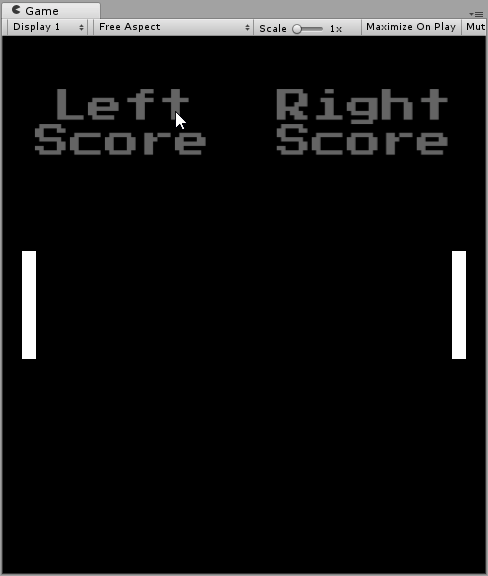# Can you override the physics behaviour for a particular object?

I’m currently trying to make a simple pong game using the built in physics. I’ve made a script that changes the velocity and direction of the ball when hitting the paddle. It works as long as the ball hits the right or left side of the paddle, but when the ball hits the bottom or top of the paddle It seems like the ball recives both the instructions of my script, but also inherits the speed of the paddle and moves a lot faster than intended. So I’m wondering if there is a way to disable the normal physics of the ball when hitting the paddle so that my script is the only thing modifying the velocity. I’ve tried using triggers, but this only resulted in the ball flying trough the paddle when at higher speeds.

Here is my code. The OnCollisionEnter2D is the interesting part. That is where I change the direction and velocity of the ball. My idea was that depending on where you hit the paddle the ball goes in a different direction. If it hits the top half the ball goes up, if it hits the bottom part the ball goes down. I also made the ball speed up every time the ball hits the paddle.

``````using System.Collections;
using System.Collections.Generic;
using UnityEngine;

[RequireComponent(typeof(Rigidbody2D))]
[RequireComponent(typeof(BoxCollider2D))]
public class Player : MonoBehaviour {

public enum Players {Player1, Player2}
public Players player = Players.Player1;
public float range = 120;
public float speed = 2;

float movementInput = 0;

Rigidbody2D rb2d;
BoxCollider2D boxCollider;

void Start() {
rb2d = GetComponent<Rigidbody2D>();
boxCollider = GetComponent<BoxCollider2D>();
}

void Update () {
GetInput();
}

void FixedUpdate() {
Move();
}

void GetInput() {
movementInput = Input.GetAxisRaw("Vertical");
}

void Move() {
if(movementInput != 0)
{
float yVel = movementInput * speed;
rb2d.velocity = new Vector2(0, yVel);
} else
{
rb2d.velocity = Vector2.zero;
}
}

private void OnCollisionEnter2D(Collision2D collision) {
{
Rigidbody2D ball = collision.gameObject.GetComponent<Rigidbody2D>();
Vector2 dir = ball.transform.position - transform.position;
float lerpVal = 0.5f + (dir.y / transform.localScale.y);
Quaternion newDir = Quaternion.Lerp(Quaternion.Euler(0, 0, -range / 2), Quaternion.Euler(0, 0, range / 2), lerpVal);
float ballSpeed = ball.gameObject.GetComponent<Ball>().speed += speedAddition;
Vector2 newVel;
switch (player)
{
case Players.Player1:
newVel = (newDir * Vector2.right) * ballSpeed;
break;
case Players.Player2:
newVel = (newDir * Vector2.right) * ballSpeed;
newVel.x = -newVel.x;
break;
default:
newVel = Vector2.zero;
break;
}
ball.velocity = newVel;

// Do cool animation or sum ting
Debug.Log("(Player) Boing");
}
}
}
``````

Just in case. Here is the code for the ball.

``````using System.Collections;
using System.Collections.Generic;
using UnityEngine;

[RequireComponent(typeof(Rigidbody2D))]
public class Ball : MonoBehaviour {

public bool goRightOnStart = true;
public float speed = 1;

Vector2 direction = Vector2.right;

Rigidbody2D rb2d;

void Start() {
rb2d = GetComponent<Rigidbody2D>();

if (!goRightOnStart)
direction = Vector2.left;
}

public void Activate() {
rb2d.velocity = direction * speed;
}

private void OnCollisionEnter2D(Collision2D collision) {
{
// Tell Manager to start new round
Manager.EndRound(transform.position.x > 0);
Destroy(gameObject);
}
}
}
``````

I need help to somehow get rid of this weird behaviour when hit I the ball on the top or bottom of the paddle. Any ideas?If you want to completely override physics, disable the rigidbody. Otherwise the rigidbody will affect how the ball moves